Latest Banking jobs   »   Reasoning Ability Quiz For SBI Clerk...

# Reasoning Ability Quiz For SBI Clerk Prelims 2022- 29th April

Directions (1-3): Study the following information carefully to answer the given questions.
Seven people i.e., Q, W, E, R, T, Y and U go to a shopping mall. All of them have different weights. Q’s weight is less than T and more than E. E’s weight is more than W but less than Y. Not more than two people are heavy than Y. T’s weight is more than Y. U’s weight is more than R whose weight is less than W. Not more than one person’s weight is less than U’s weight. E is lighter than Y. Q’s weight is not less than Y.

Q1. Who among the following is fourth heaviest?
(a) Y
(b) W
(c) E
(d) Q
(e) U

Q2. If there is one more person H, whose weight is more than E but less than Q. How many people go to shopping mall between H and U?
(a) Three
(b) Two
(c) Four
(d) Either three or two
(e) Five

Q3. If W’s weight is 49 kg, and third heaviest person’s weight is 55kg, then what is the possible weight of E?
(a) 53
(b) 59
(c) 42
(d) 35
(e) 65

Directions (4-5): Study the information carefully and answer the questions accordingly.
There are six students in a class with different weights. The weight difference of V and W is 19Kg. The weight of R is not an odd number. Y is lighter than M but heavier than R. W is heavier than V and lighter than S. The weight of W is 12Kg more than Y. The weight of the second lightest person is 51kg. Only three people are lighter than M.

Q4. How many people are lighter than W?
(a) Two
(b) Four
(c) One
(d) Three
(e) None of these

Q5. What could be the weight of M?
(a) 72kg
(b) 65kg
(c) 56kg
(d) 53kg
(e) 71Kg

Directions (6-8): Study the following information carefully and answer the given questions.
Amongst six friends – P, Q, R, S, T and U, each has different heights. P is taller than Q but not as tall as U. S is shorter than only two persons. U is not the tallest person. T is shorter than R. Q is not the shortest person.

Q6. Who among the following is the second tallest person?
(a) R
(b) U
(c) P
(d) T
(e) None of these

Q7. If the height of S is 165 cm, then what may be possible height of Q?
(a) 167 cm
(b) 166 cm
(c) 163 cm
(d) 168 cm
(e) 169 cm

Q8. How many persons are shorter than P?
(a) One
(b) None
(c) Three
(d) Two
(e) Four

Directions (9-11): Study the following information carefully and answer the given questions.
Amongst six friends – F, G, H, I, J and K each have different heights. G is not as tall as J but taller than F. H is shorter than K but taller than J and F. I is the third shortest among all the friends. The height of the tallest person is 40cm and the second shortest person is 21cm.

Q9. Who is just shorter than G?
(a) J
(b) H
(c) F
(d) K
(e) None of these

Q10. If total height of G and J is 56cm, then what is the height of J?
(a) 38cm
(b) 42cm
(c) 18cm
(d) 16cm
(e) 35cm

Q11. How many persons are taller than K?
(a) One
(b) None
(c) Three
(d) Two
(e) Four

Directions (12-13): Study the following information carefully and answer the following questions.
Six people i.e. A, B, C, D, E, and F have different heights. A is taller than B but not as tall as F. C is shorter than only two persons. F is not the tallest person. B is not the shortest person. E is shorter than D. The height of the second shortest person and the second tallest person is 151 cm and 183cm respectively.

Q12. How many people are shorter than A?
(a) Three
(b) One
(c) Two
(d) Four
(e) None

Q13. What should be the possible height of E?
(a) 153 cm
(b) 198 cm
(c) 145 cm
(d) 163 cm
(e) 152 cm

Directions (14-15): Study the following information and answer the given questions.
Eight people L, M, N, O, P, Q, R, and S have different weights. P is just heavier than Q who is heavier than S. L is the lightest person in the group. M is just heavier than O but just lighter than R. Only two people are lighter than O. N is not the heaviest. S is not just heavier than L.

Q14. Who among the following is the heaviest?
(a) Q
(b) R
(c) P
(d) M
(e) None of the above

Q15. Who among the following is just lighter than S?
(a) M
(b) R
(c) O
(d) L
(e) None of the above

Solutions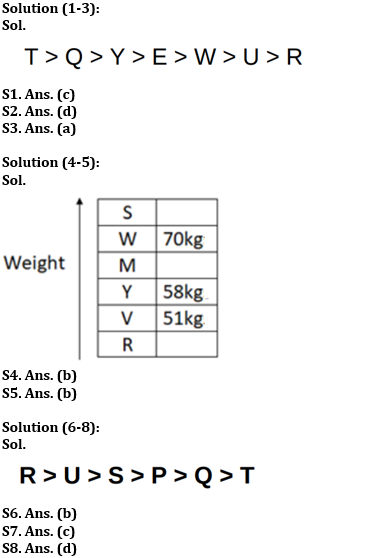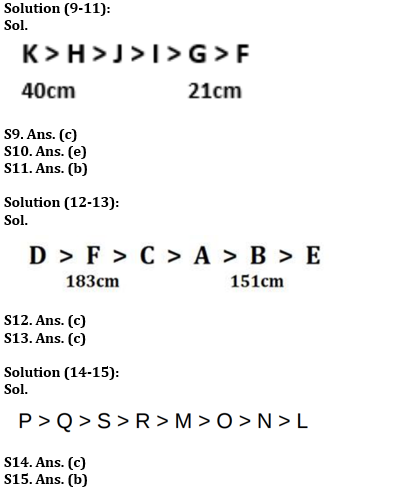#### Congratulations!Download Hindu Review of October 2021: Free PDFIncorrect details? Fill the form again here

Download Hindu Review of October 2021: Free PDF

Thank You, Your details have been submitted we will get back to you.
•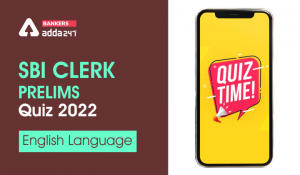English Quizzes For SBI Clerk Prelims 20...
•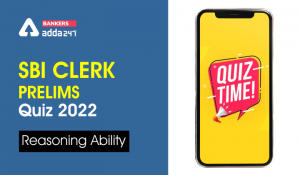Reasoning Ability Quiz For SBI Clerk Pre...
•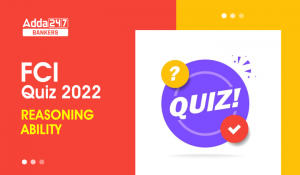Reasoning Ability Quiz For FCI Phase I 2...
•English Quizzes For SBI Clerk Prelims 20...
•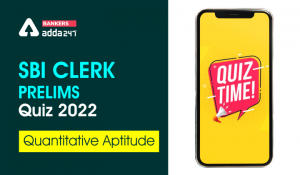Quantitative Aptitude Quiz For SBI Clerk...
•Reasoning Ability Quiz For IBPS RRB PO C...